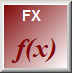Digital F(x) Function Block

The digital F(x) function block will allow you to derive a value from an arbitrary mathematical formula involving any output from the device's existing function blocks. You can mix analog and digital values liberally. A digital value used in an analog context has the value 1.0 (On) or 0.0 (Off), and an analog value used in a digital context is On (value != 0.0) or Off (value = 0.0).

The output of the block is a digital value, On (value != 0.0) or Off (value = 0.0). Since the blocks output value is digital it uses less memory and frees up space for other analog blocks.

Digital F(x) definition parameters

 Parameter Description Name Function block name, see common parameters Formula The mathematical formula to compute the block's output value.

The  mathematical formula can contain the following operators:

Mathematical Operators

 Operator Description + Add - Subtract * Multiply / Divide

Comparison Operators

 Operator Description > Greater Than < Less Than >= Greater Than or Equal <= Less Than or Equal = Equal

Boolean Operators

 Operator Description ! not NOT & and AND | or OR

Operator Precedence

Operator precedence is divided into three groups as shown below. Multiple operators from the same group are evaluated left to right. You can use parenthesizes liberally to force a particular evaluation order as required.

 Precedence Operators Highest !  () *  /  & Lowest +  -  >  <  >= <= = |

Supported functions

 Operator Description dt() The elapsed time interval, in seconds, since last iteration sin(x) Trigonometric SIN function x is in radians cos(x) Trigonometric COS function x is in radians sqrt(x) Square root min(x,y) lesser of  x or y max(x,y) greater of  x or y mod(x,y) Floating point modulus, remainder of x / y exp(x) returns the exponential function of its argument; i.e., ‘e’ to the power of ‘x’. - MBus_io12_LCD & MBus_io14_DIN only log(x) returns the natural logarithm of 'x' - MBus_io12_LCD & MBus_io14_DIN only pow(x,y) returns x raised to the power of y (x^y) - MBus_io12_LCD & MBus_io14_DIN only